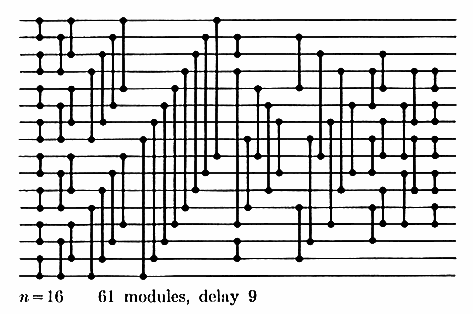### Subscribe

E-mail:

Jethro Beekman on
Technology & Policy

## Parallel Nibble Sort

by Jethro Beekman – Jan 28, 2015 – Updated: Jul 20, 2015. Filed under: English, Technology, SIMD.

Update July 20, 2015: The winning solution by Alexander Monakov also uses a sorting network but transposes the items to be sorted to sort 32 nibbles in parallel with a length 60 network, instead of my 4 nibbles with a depth 9 network. Hans Wennborg has a nice write-up of that solution.

Professor John Regehr at University of Utah held a small programming contest for “nibble sort”. The goal is to sort nibbles in a 64-bit value, 1024 times, as fast as possible. For example, the nibble sort of `0xbadbeef` is `0xfeedbba000000000`.

### Algorithm

I chose to implement the sort using a sorting network. I used the following minimum-depth network to sort 16 items, which was designed by David C. Van Voorhis.Figure 1. From “The Art of Computer Programming, Volume 3”.

The inputs are distributed in rows on the left, and each vertical line segment compares the two numbers on the parallel lines, swapping them if the upper element is less than the lower element.

### Parallelization

For each of the 9 stages, the elements to be sorted are split into two buckets, and the same indices in each bucket are compared and potentially swapped at the same time using SIMD instructions. The split for each stage is a different permutation depending on which elements are to be compared. This process was inspired by the paper “Efficient implementation of sorting on multi-core SIMD CPU architecture”. After each stage, the buckets are then again combined into a single list using the inverse permutation. The permutations of combining the buckets from the last stage and splitting them again for the next stage can be reduced to a single permutation.

```function sort(input):
b1:b2 <- input
for i := 1 to 9:
b1:b2 <- each_min(b1,b2):each_max(b1,b2)
b1:b2 <- permute(step=i,b1:b2)
return b1:b2
```

Algorithm 1. Parallel network sort.

### Implementation

On IA-32, the smallest unit that can be processed is a byte. Every 2 of the 16 nibbles in the input word are unpacked into the lower nibble of 2 bytes for a total of 16 bytes to be sorted, and each bucket is 8 bytes. AVX2 can process 32 bytes or 4 buckets in parallel. This implementation runs more than 72× faster than the reference implementation on my test machine.

### Discussion

Currently, the min/max operation takes ½ operation per word, while the permute operation takes 1½ operations per word. The reason the permutation requires so many instructions is that both buckets need to be in the same register for the permute operation but they need to be in seperate registers for the min/max operation. By carefully considering the shuffling constants, it’s possible to do permutations #3 and #8 in 1 operation per word and ½ operation per word, respectively.

The application can be further sped up by using multiple threads to do the sorting, each of the 1024 elements can be sorted individually. When working with much larger datasets, subsets of it can still be sorted individually, so this algorithm scales very well.